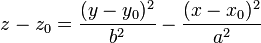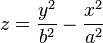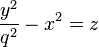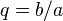# Difference between revisions of "Hyperbolic paraboloid"

Jump to: navigation, search

## Definition

The surface type is not unique up to isometry or even up to similarity transformations, but rather, depends on multiple nonzero parameters$a,b$. If we're considering the surface up to rigid isometries, the parameters are unique. If we're considering the surface up to similarity transformations, the parameters are unique up to projective equivalence.

The surface, however, is unique up to affine transformations, which include transformations that do not preserve the affine structure.

### Implicit and parametric descriptions

Degree of generality Implicit description What the parameters mean Parametric description What the additional parameters mean Comment
Arbitrary Fill this in later This version need not be centered at the origin and need not be oriented parallel to the axes.
Up to rotations$z - z_0 = \frac{(y - y_0)^2}{b^2} - \frac{(x - x_0)^2}{a^2}$
Up to rigid motions (rotations, translations, reflections)$z = \frac{y^2}{b^2} - \frac{x^2}{a^2}$
Up to similarity transformations$\frac{y^2}{q^2} - x^2 = z$ Here$q = b/a$ and we've done a similarity transformation.
Up to all affine transformations (not permissible if we want to study geometric structure)$y^2 - x^2 = z$